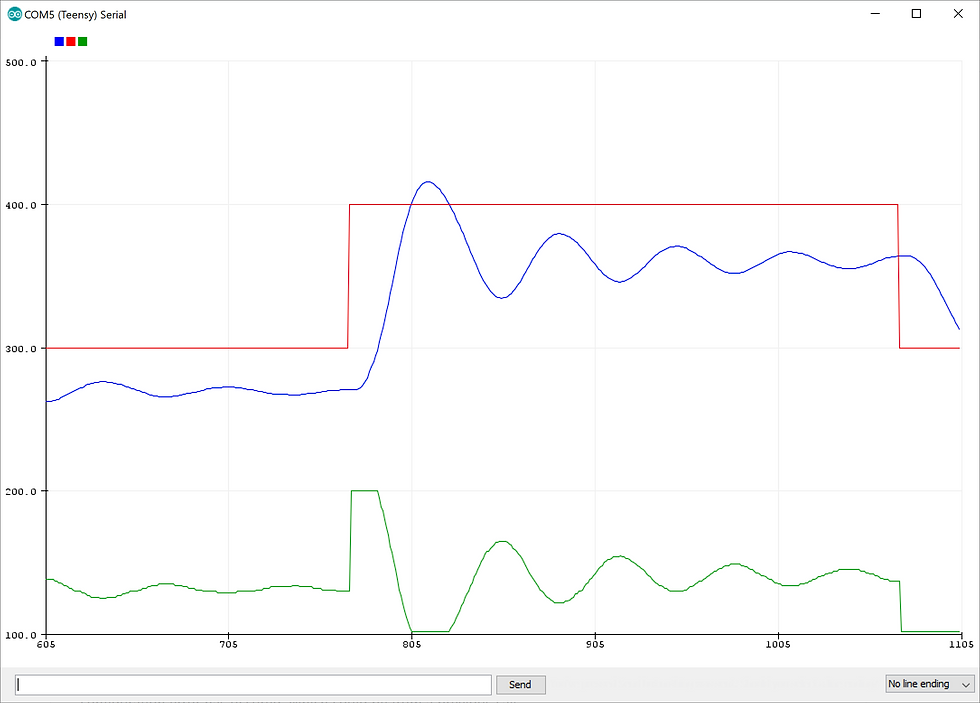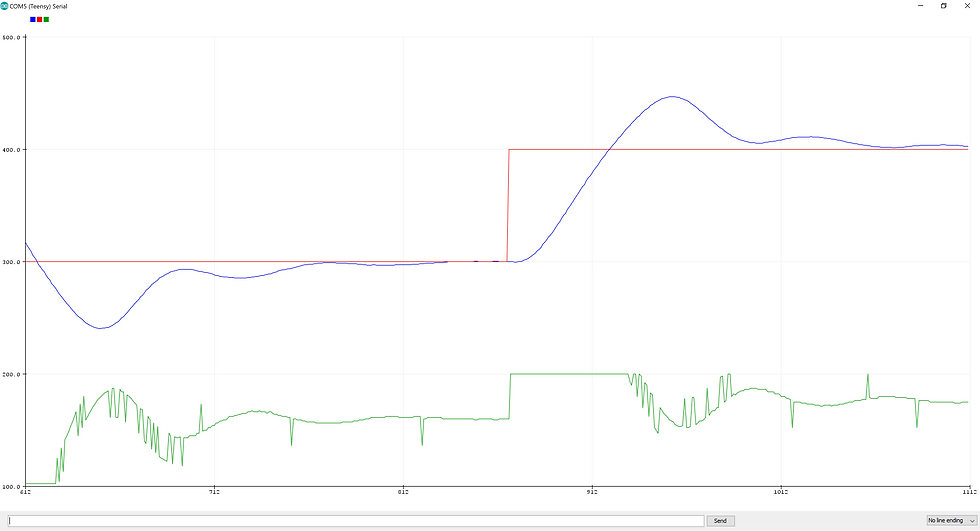top of page
Search

# PID Tuning for Catheter Reflow HeaterWhat if you needed very precise heat control for your catheter reflow process and commercial solutions weren't cutting it? Maybe you need to reflow different polymers at different temperatures along the length of your device. What do you do? Maybe creating your own PID controller and tuning it is the answer. This article will walk you through a tuning methodology.The Basics: We'll assume that you're familiar with PID control but let's go over some basics. The purpose of a PID controller is to reduce the error between a process set point and the actual process parameter. This difference is called the error or "e" in the equation. This error is what drives the whole control loop.

The "P" coefficient is multiplied by the error to affect the output. The higher the error, the more the proportional term reacts. The "I" coefficient is multiplied by the integral of the error over time. Simply put, this term grows over time the longer there is error in the system. Finally, the "D" coefficient is multiplied by the derivative of the error over time. How fast the error is changing affects this term.

Setting a goal. It's important to define what an acceptable outcome will be. If you don't define the goal, you may never finish the tuning process. In this case we want the process temperature to reach the target temperature in 10 seconds or less. We want to limit overshoot to less than 10º C and we want the process temperature to stay within 5º C of the target.

Seeing the Process. It can be daunting to think of where to start tuning a PID system. The first thing you need is a way to visualize the process parameters and change the process setpoint. Here we've programmed the heater to alternate the target temperature between 300º C and 400º C (red line). We're plotting the actual air temperature in blue. The heater output is shown in green and varies between 0-100% power.

Is the Process Controllable? This may sound like a stupid question but answering this upfront can save you countless hours and frustration. If you were to turn on the heater element, could the temperature reach and surpass the target? Does it come down with the heater off? If the heater doesn't have enough power to reach the target, or has way too much power and blows past the target, you'll never be able to control the system. (I've learned this the hard way). The heat should also react proportional to the power applied to the heater. Say the first 80% of power commanded did nothing, and then suddenly the heater turns full on at 81% commanded power. This would make controlling the system impossible.P=10, I=0, D=0P=20, I=0, D=0

Adjusting the P coefficient. The first thing to do is set the I and D coefficients to zero and start adjusting the P. The images above shows a P at 10 and 20. When P is set to 20, there are large oscillations in the temperature. With P set to 10, the Oscillations are dissipating quickly. We could pick a P term that doesn't produce any Oscillations or overshoot, but we will take care of a little overshoot later with the D coefficient. Notice that in either case, the temperature is consistently under the target. This will be resolved with the I coefficient.P=10, I=1, D=0

Adding I coefficient. Now that we have the P coefficient producing some output, we can add I to the controller. With I set to 1 in this case, you can see that already the temperature is running much closer to the target. Some of the oscillations from the P term are reduced as a result of the smaller error too.P=10, I=3, D=4

Adjusting the D coefficient. Now that P and I are set up, we can increase the D coefficient and attempt to remove overshoot and oscillations. The image above shows the response after adjusting the D coefficient. Notice the extra I term keeps the temperature very close to the target. But there is too much overshoot and there are still some oscillations.P=5, I=1, D=5

Refining. In this image, we see a much more predictable response. Overshoot is reduced and the process quickly finds the target without oscillating. The overshoot and oscillations were reduced by reducing the P and I coefficients. As we saw from the initial P adjustment, this term was producing some oscillations. It was too much to fix with the D term, so we backed off of the P.P=4.5, I=0.7, D=5

Finishing up. With a little more refinement, we have a process that quickly reaches the target temperature without overshoot. This type of controlled response is critical when changing temperatures along the length of a catheter. In this extreme example, the temperature setpoint changes 100º C and the process temperature follows in less than 10 seconds